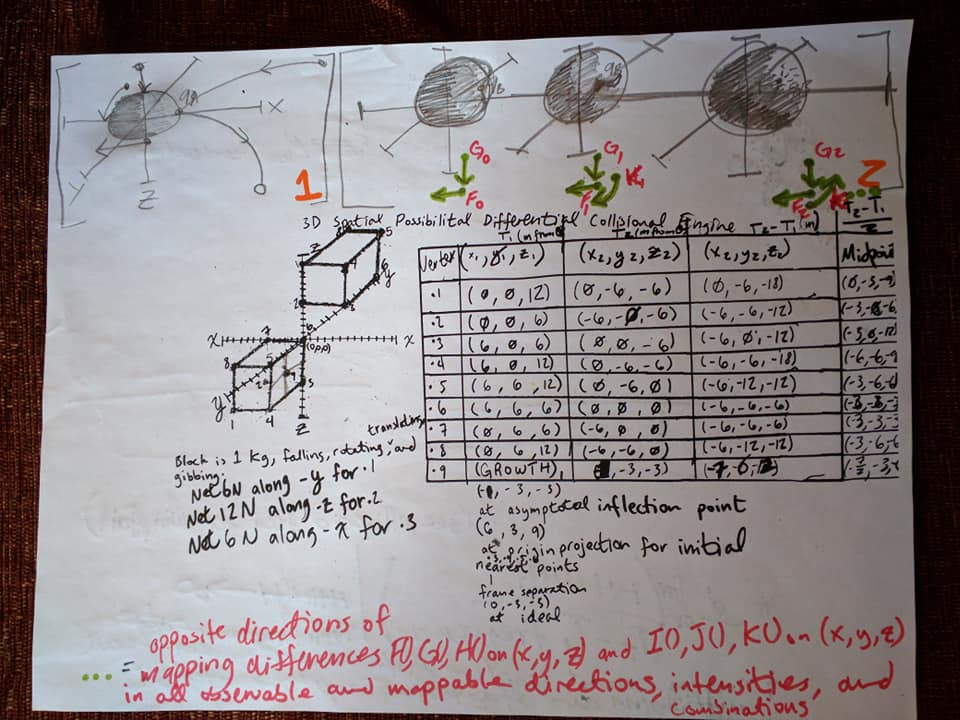## Juggling Work and LifeThere are three objects rotating around a center point, sitting a good distance away from three other objects rotating around a different center point. This proof is to calculate exactly the force, velocity, distance, and time necessary to move one of the objects A, B, or C, into one of the other objects D, E, or F.

You can use differences at any given time to find the distance and predict position of one of the objects.

You can use trigonometry (Sine=Opposite/Hypotenuse, Cosine=Adjacent/Hypotenuse, Tangent=Opposite/Adjacent, (sq(A) + sq(B) = sq(c)), et cetera) to find the angle for the path between object (A, B, or C) and (D, E, or F). With a path angle, you simply wait one harmonic cycle (About the time it should take for both circles of objects to be in the same position again), and then, by calculating the time and velocity over a certain distance of a test projectile, with Velocity=Distance x Time, where distance is equal to the difference between the position of object (A, B, or C) at Time 1, and (D, E, or F) at Time 2, time 1 is equal to the time at ejection of projectile, and Time 2 is equal to time at projectiles arrival at position of (D, E, or F) in the other juggling circle.

All that is left is to calculate:
1) The force of the incident object that pushes the ball required to produce this movement, with (Force1 = Mass1 x Acceleration1) + (Force 2 = Mass2 x Accelleration2) = (1/2 x Mass2 x d((Velocity2)/dx))
2) The deformation and change to center of gravity of the ball (A, B, or C) in juggling circle 1, after being pushed out of juggling circle 1, and the deformation and change to center of gravity of the ball (D, E, or F) in juggling circle 2, as it is struck by the projectile.

Some have more experience modelling 3 Dimensional floating orbs physically and mathematically in relation to range, to generate interactions.

« Previous: Mostly Memories, Maybe Mine

This site uses Akismet to reduce spam. Learn how your comment data is processed.